Nyuk Khui, P. L., Rahman, M. R., Kuok, K. K., Bin Bakri, M. K., Adamu, M., Tazeddinova, D., Kazhmukanbetkyzy, Z. A., and Torebek, B. (2021). "Small-size jatropha seed biochar extracted from microwave pyrolysis: Optimization of its biocomposites mechanical properties by mixture design," BioResources 16(3), 4716-4730.

#### Abstract

Microwave pyrolysis of finely ground jatropha seed biochar was used as bio-filler to develop biocomposites. Effects influencing the mechanical properties of the biocomposites were investigated based on varied material ratio. Ratios by percentage of weight were determined by D-optimal (custom) mixture design using the Stat Ease “Design Expert”. The mechanical properties, such as tensile strength, modulus of elasticity, and microhardness, were the dependent variables (response). Bio-filler content was optimised to attain the overall best mechanical properties for the biocomposites. The optimized biocomposite that showcased good tensile strength, modulus of elasticity, and microhardness biocomposite ratio’s predicted mechanical properties mean values were tensile strength (9.53 MPa), modulus of elasticity (0.730 GPa), and microhardness (20.4 HV) for polylactic acid and biofiller mixture; and tensile strength (7.92 MPa), modulus of elasticity (0.668 GPa), and microhardness (18.7 HV) for polylactic acid, biofiller, and poly(ethylene-alt-maleic anhydride) mixture. Models generated by the mixture design showcased some degree of noise and error present; however, the outcome through the optimization step was generally reliable for predicting the mechanical properties. Additional data gathered through experimental testing and replicates could improve the reliability of the model.

Small-size Jatropha Seed Biochar Extracted from Microwave Pyrolysis: Optimization of Its Biocomposites Mechanical Properties by Mixture Design

Perry Law Nyuk Khui,a Md. Rezaur Rahman,a,* Kuok King Kuok,b Muhammad Khusairy Bin Bakri,a Muhammad Adamua, Diana Tazeddinova,c Zhumayeva A. Kazhmukanbetkyzy,d and Baibatyrov Torebek d

Microwave pyrolysis of finely ground jatropha seed biochar was used as bio-filler to develop biocomposites. Effects influencing the mechanical properties of the biocomposites were investigated based on varied material ratio. Ratios by percentage of weight were determined by D-optimal (custom) mixture design using the Stat Ease “Design Expert”. The mechanical properties, such as tensile strength, modulus of elasticity, and microhardness, were the dependent variables (response). Bio-filler content was optimised to attain the overall best mechanical properties for the biocomposites. The optimized biocomposite that showcased good tensile strength, modulus of elasticity, and microhardness biocomposite ratio’s predicted mechanical properties mean values were tensile strength (9.53 MPa), modulus of elasticity (0.730 GPa), and microhardness (20.4 HV) for polylactic acid and biofiller mixture; and tensile strength (7.92 MPa), modulus of elasticity (0.668 GPa), and microhardness (18.7 HV) for polylactic acid, biofiller, and poly(ethylene-alt-maleic anhydride) mixture. Models generated by the mixture design showcased some degree of noise and error present; however, the outcome through the optimization step was generally reliable for predicting the mechanical properties. Additional data gathered through experimental testing and replicates could improve the reliability of the model.

Keywords: Composite; Biochar; D-optimal mixture design; Mechanical properties

Contact information: a: Faculty of Engineering, Universiti Malaysia Sarawak, Jalan Datuk Mohammad Musa, Kota Samarahan, Sarawak 94300 Malaysia; b: Faculty of Engineering, Computing and Science, Swinburne University of Technology Sarawak Campus, Jalan Simpang Tiga, Kuching, Sarawak 93350 Malaysia; c: Faculty of Engineering, South Ural State University, 76, Lenin Prospekt, Chelyabinsk 454080 Russia; d: Zhangir Khan West Kazakhstan Agrarian – Technical University, Zhangir Khan St 51, Uralsk 090009 Kazakhstan; *Corresponding author: rmrezaur@unimas.my

INTRODUCTION

Microwave pyrolysis of jatropha seeds has been used in a few studies to produce biochar (Das et al. 2018; Law et al. 2021). The pyrolysis extraction tool produced good oil yields, particularly from jatropha seeds, suggesting that it could be used as a biodiesel fuel (Kanaujia et al. 2016; Sugumaran et al. 2017; Ruggiero et al. 2019). The excess or remaining material after pressing a larger quantity of oil from the fruits and nuts is sometimes poisonous and must be chemically treated before being used as agricultural feedstock or dumped in landfills (Ruggiero et al. 2019). The use of Jatropha curcas L. fruits and seeds as an alternate source of ecological biodiesel and possible filler in composites was motivated by advanced technologies (Law et al. 2021). Ball milling processes were used to successfully synthesise and characterise microwave pyrolysis jatropha seed biochar into very small size bio-filler (Law et al. 2020). As a result, the mechanical properties of biocomposites using the microwave pyrolysis jatropha seed biochar as bio-filler and biodegradable polymer matrix were optimised in this study.

The majority of biodegradable PLA biocomposites have shown high mechanical properties, such as cellulose microcrystalline fibres and nano-whiskers, carbon, wood fibres, and flour (Petersson et al. 2007; Rahman et al. 2021; Belhamdi et al. 2016). Wood, kenaf, bamboo, and hemp are the most common fibres or fillers used to incorporate in the PLA in biocomposites (Pan et al. 2007; Nyambo et al. 2010; Rahman et al. 2021). In comparison to neat PLA, most of the PLA biocomposites modulus was raised, and elongation at break was decreased (Chun et al. 2020). Carbon fibres or fillers, both natural and man-made, have been found to improve the mechanical properties of PLA-based composites (Li et al. 2020). In terms of developing a composite with good tensile strength, numerous studies have employed laminates and continuous unidirectional oriented reinforcements to produce composites with higher tensile strength (McNally 2008; Yang et al. 2016; Hazer et al. 2018; Rajak et al. 2019). However, the bio-filler utilized is considered as micro-nano particles reinforcements; hence, the composite is a particle reinforced composite. An improved hardness, wear resistance, and fracture toughness are the probable outcome (Ghalia and Abdelrasoul 2018; Rajak et al. 2019).

To optimise the bio-filler ratio and material usage with respect to the biocomposite mechanical properties, a design of experiments program by StatEase called “Design Expert” was used. Design of experiments is a statistical technique to evaluate the effects of linear, non-linear, and independent variables (e.g., bio-filler, polymer matrix) on dependent variables (mechanical properties) (Keinänen et al. 2018; Venkatesan et al. 2019; Zaib et al. 2019). The D-optimal (custom) mixture design uses the number of input variables to be one. Therefore, the measured response in the mixture configuration is independent of the volume of the mixture and depends only on the iterations of the components in the mixture (Hare 1991). Optimal modelling algorithms test the unbiased method parameter predictions for the lowest number of experimental runs in a mixture configuration (Jeirani et al. 2012; Varanda et al. 2017).

The response-dependent variables are the tensile strength, elasticity modulus, and Vickers microhardness. The significance of using this program is to predict and determine the optimum ratios for fabrication in favour of the best mechanical properties possibly achievable. For a simple bio-filler-to-matrix composite mixture, a D-optimal (custom) mixture design option was utilised. The program can simulate data that shows the optimum ratio, dependant to which parameter is desired to be maximised or minimised. As presented by StatEase and other researchers, the D-optimal (custom) mixture design approach used for response analyses and optimisation will be according to the standard protocol, so results may be comparative to other studies (Zarrinbakhsh et al. 2019; Radfar et al. 2020; Visetvichaporn et al. 2020).

Arrigo et al. noted that biochar-reinforced polylactic acid (PLA) biocomposites can be tailored to meet commercial specifications by optimising the biochar filler content and the suitable processing method. The researcher’s findings present biochar as a suitable replacement for conventional fillers (i.e., carbon black, talc, and CaCO3). Hence, expanding the applicability of biochar and its biocomposites to applications such as building, packaging, automotive, and electronics (Das et al. 2018; Arrigo et al. 2020). Moreover, the low cost of production of bio-filler, combined with the ability to extract it from various waste sources, reflects an added benefit to the circular economy solution (Arrigo et al. 2020).

EXPERIMENTAL

Materials

Bio-filler reinforcements used for biocomposite fabrication were synthesized based on the previous work by Law et al. (2020). The 30 hrs ball milling filler was used. Polylactic acid (PLA) and poly(ethylene-alt-maleic anhydride) were supplied by Universiti Malaysia Sarawak (UNIMAS) (Kota Samarahan, Sarawak, Malaysia). A hydraulic molding press (GT-7014-A50C; GOTECH, Taichung City, Taiwan) was used, which has the capability of a dual functioning hot and cold pressing operation for biocomposite fabrication. A universal testing machine (MSC-5/500; Shimadzu, Kyoto, Japan) was used to conduct the tensile test for numerous mechanical properties from the biocomposite samples. A Vickers micro hardness tester (HMW-G21; Shimadzu, Kyoto, Japan) was used to conduct the microhardness test for the biocomposite samples. Most of the experimental testing was conducted at the chemical engineering department of Universiti Malaysia Sarawak (UNIMAS) (Kota Samarahan, Sarawak, Malaysia).

Methods

“D-optimal (custom) mixture design” with Design Expert software

A biocomposite ingredient mixture ratio, response analysis, and optimization were designed with software by StatEase called Design Expert (version 11, Minneapolis, MN, USA). Within the “Standard Designs” for Design Expert, D-optimal (Custom) Design was selected under the category “Mixture”. This lets the user produce an array of filler and matrix content ratios, prior to biocomposite fabrication. A standard number of replicates and runs was selected to the minimum standard under the software guidelines. The following abbreviations were used for the ingredients in the mixture design and testing:

• PLA = Polylactic Acid
• PLA/PEMA = Polylactic Acid + Poly(ethylene-alt-maleic anhydride)
• PLA/BC = Polylactic acid + Bio-filler
• PLA/PEMA/BC = Polylactic Acid + Poly(ethylene-alt-maleic anhydride) + Bio-filler

Mechanical tensile strength test for biocomposites

The tensile strength test was conducted with a maximum loading capacity of 300 kN and a crosshead speed of 0.7 mm/min. A total of 5 runs were conducted for each sample ratio variation to attain an average value, according to the ASTM D638-14 (2014) testing standard TYPE IV, with a crosshead speed 5mm/min.

Vickers microhardness test for biocomposites

A Vickers indenter with squared-based pyramidal-shaped diamond indenter with face angles of 136° was used during the test. The force was setted at HV 0.3 (2.942 N), and the indenter was held for 10 s. A total of 5 runs were conducted at different locations on the biocomposite to attain an average (HV) value, as per ASTM E384-17 (2005). The following calculation represent the Vickers microhardness formula (HV) equivalent to the units (N/mm2) in Eq. 1,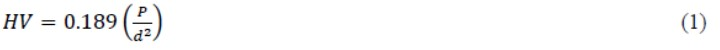where P is expressed in Newton units (N), and d is the average length of the diagonal left by the indenter (mm).

RESULTS AND DISCUSSION

Through comparing the bio-filler performance, it was observed that the overall tensile strength (TS) of the biocomposites did not improve with the increase in bio-filler content (Table 1). The interfacial bonding between polymer matrix and reinforcement were poor with high bio-filler content, and it was recommended to undergo modification of bio-filler, such as surface functionalization (physical or chemical) (Poulose et al. 2018). A study shows a similar effect with the introduction of biochars, which allows the composite to sustained similar tensile strength as the control, with additional properties such as improved electrical conductivity and thermal degradation (Nan et al. 2015).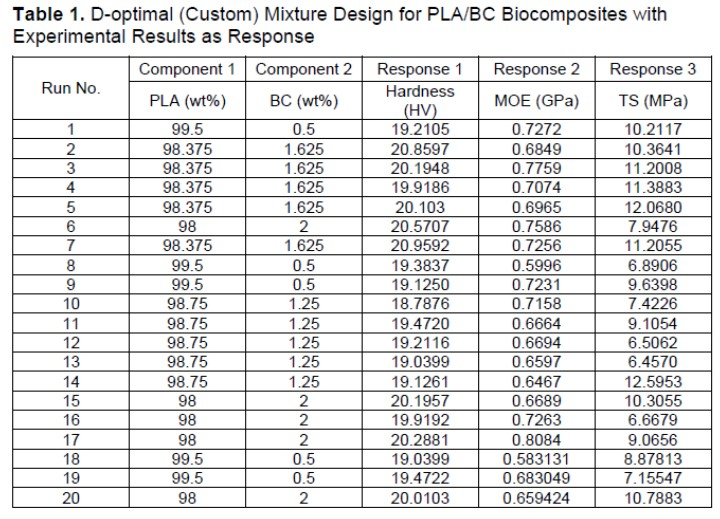The modulus of elasticity (MOE) for the PLA/BC biocomposite and their iterations showed slight improvements. The iterations for PLA/PEMA/BC had a similar MOE range as the iterations for PLA/BC. The increased MOE can be attributed to the brittle behaviour of the biocomposite and affected the hardness, as well. These results were comparable to numerous studies, where biocarbon was used for reinforced polymer composites, whereas the tensile strength seemed to be maintained within the performance of the control samples with improved MOE (Nan et al. 2015; Poulose et al. 2018).

The PLA/BC increased in hardness as the bio-filler content increased. The high value of hardness of all samples was at 1.625 wt% for PLA/BC with a value of 21.0 HV. A similar study reported the usage of multi-wall carbon nanotubes (CNT) (0.2 wt%) in epoxy resin without surface modification and with an average microhardness 21.7 HV, whereas the control epoxy resin was 21.3 HV. In addition, the surface modification on the CNT fillers enabled the polymer composite to achieve a microhardness value of 28.0 and 29.6 HV (Franceschi et al. 2015).

The results of the mechanical test results and filter-to-matrix content for PLA/BC biocomposites are presented in Table 1. In total, there were 20 runs with replicates for each iteration (0.5, 1.25, 1.625, and 2.0 wt%). The response are individual mechanical test results for each sample, rather than an average value. The replicates increased the number of data points for the model to plot and produced the predicted values. The average values for the control samples mechanical properties were as follows: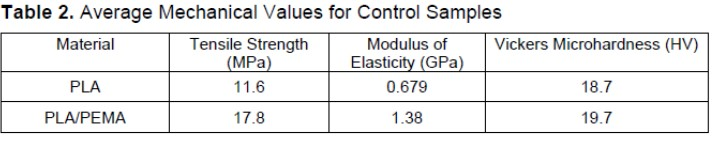Table 3 shows that analysis of variance (ANOVA) utilized a quadratic model, which was a best fit for all responses in the mixture design for PLA/BC. However, the coded equation with variables A and B were the coding factors by design expert mixture design. Table 4 shows the tabulated diagnostic results of the predicted values versus the actual experimental values. In each run, the actual versus predicted value of TS (MPa) were varied in comparison to the values for hardness (HV) and MOE (GPa). Hence, the diagnostic results did correlate with the ANOVA results where it showed the model had a significant percentage that the predicted value in relation to the actual value were occurring due to noise.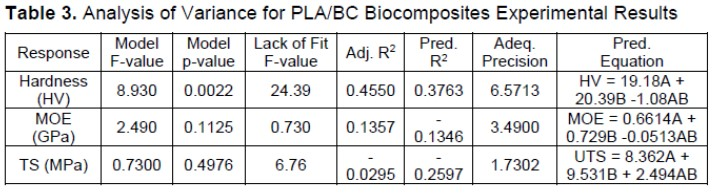The ANOVA produced by the mixture design for the PLA/BC hardness (HV) response showed the model F-value of 8.930, which implied that the model was significant. There was a 0.22% chance that an F-value that large was possibly due to noise. Model p-value (0.0022) was less than 0.05, indicating the model terms were significant. The Lack of Fit F-value of 24.39 implied that the Lack of Fit is a relevant factor. According to the Design Expert based on ANOVA results, there was a 0.01% chance that the Lack of Fit F-value could occur due to noise. The predicted R² of 0.3763 was in reasonable agreement with the adjusted R² of 0.4550 because the difference was less than 0.2. Adequate precision measured the signal to noise ratio. According to Design Expert, a ratio greater than 4 was desirable. The ratio of 6.571 indicated an adequate signal. This model was used to navigate the design space.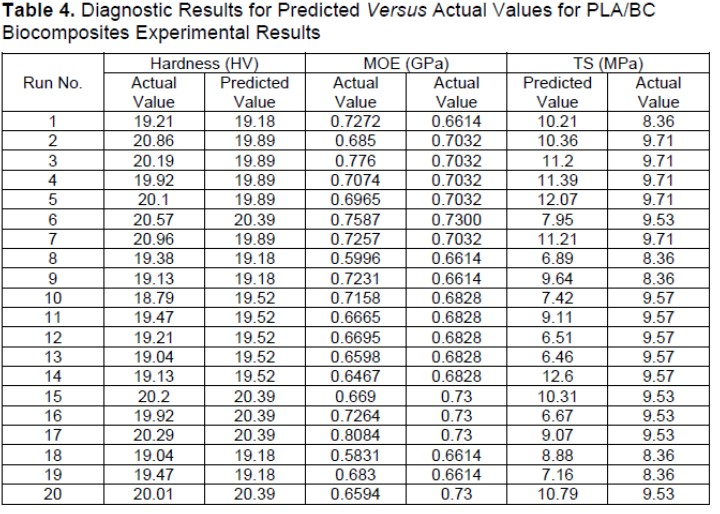The ANOVA produced by mixture design for the PLA/BC MOE (GPa) response showed that the model F-value of 2.49 implied the model was not significant relative to the noise. There was a 11.25% chance that an F-value that large could occur due to noise. The model p-value (0.1125) was greater than 0.05, hence model terms were not significant. The Lack of Fit F-value of 0.73 implied that the Lack of Fit was not significant relative to the pure error. There was a 40.55% chance that a Lack of Fit F-value that large could occur due to noise. A negative predicted R² of -0.1346 implied that the overall mean was a better predictor of the response than the current model. An adequate precision ratio of 3.49 indicated an inadequate signal to use this model to navigate the design space.

The ANOVA for the PLA/BC TS (MPa) response showed that the model F-value of 0.73 implied that the model was not significant relative to the noise. There was a 49.76% chance that an F-value that large could occur due to noise. The model p-value (0.4976) was less than 0.05, indicating that the model terms were significant to PLA/BC TS, which was directly taken from the Design Expert. The Lack of Fit F-value of 6.76 implied that the lack of fit was significant. There was only a 1.93% chance that a Lack of Fit F-value that large could occur due to noise. A negative predicted R² of -0.2597 implied that the overall mean was a better predictor of the response than the current model. Adequate precision measured the signal to noise ratio. A ratio of 1.73 indicated an inadequate signal and the model was inadequate to navigate the design space.

Similar studies utilizing D-optimal (custom) mixture design describe that the contribution of significant and not significant indications by the ANOVA influenced the model usability and reliability (Homkhiew et al. 2014; Radfar et al. 2020). Noise percentages generated by the mixture design were regarding the ANOVA values, because the least amount of noise percentage was desirable for the model. Similarly, the Lack of Fit of the model was desired to not be significant, as a fitting model with the least amount of noise was better at predicting the response, as well as minimising residuals between the predicted and response values (Homkhiew et al. 2014; Radfar et al. 2020).

The plotted graphs for the PLA/BC biocomposites predicted versus actual values generated by the mixture design are shown in Figs. 1 to 3. The HV plot for PLA/BC biocomposite shows a slightly better fitting model compared to the MOE and TS plots. Values that were closer to the linear plotted line resulted in a better model for optimisation, as the actual and predicted values had less residual value (Homkhiew et al. 2014).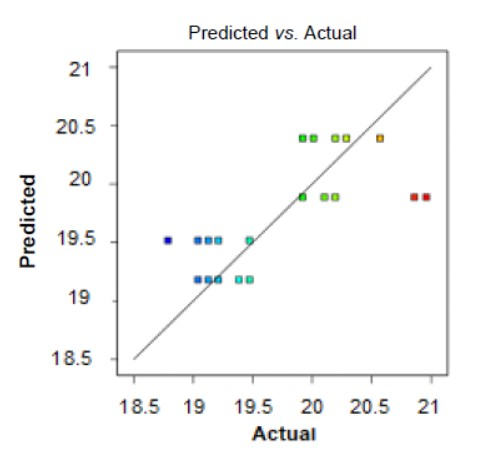Fig. 1. Predicted versus actual hardness (HV) for PLA/BC biocomposites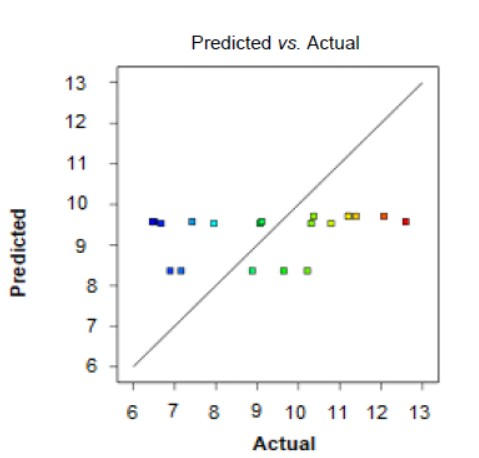Fig. 2. Predicted versus actual tensile strength (TS) for PLA/BC biocomposites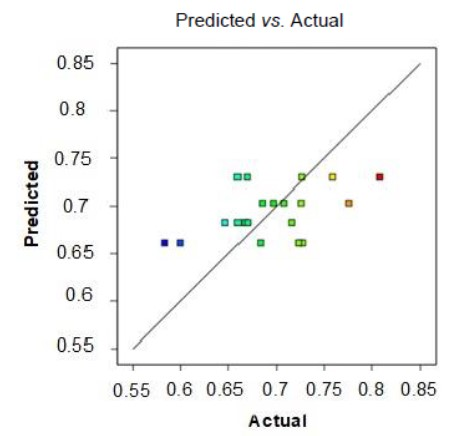Fig. 3. Predicted versus actual modulus of elasticity (MOE) for PLA/BC biocomposites

The ANOVA for the PLA/PEMA/BC hardness (HV) response showed that the model F-value of 14.29 implied that the model was significant. There was a 0.02% chance that an F-value that large could occur due to noise. The model p-value (0.0002) was less than 0.05 and indicated that the model terms were significant. The Lack of Fit F-value of 2.49 implied that the lack of fit was not significant relative to the pure error. There was a 13.42% chance that a Lack of Fit F-value that large could occur due to noise. The predicted R² of 0.4655 was in reasonable agreement with the adjusted R² of 0.5831, where the difference was less than 0.2. An adequate precision ratio of 8.522 indicated an adequate signal. This model was used to navigate the design space.

The ANOVA for the PLA/PEMA/BC MOE (GPa) response showed that the model F-value of 2.42 implied that the model was not significant relative to the noise. There was a 11.92% chance that an F-value that large could occur due to noise. The model p-value (0.1192) was greater than 0.05 and indicated that the model terms were not significant. Values greater than 0.1000 indicated that the model terms were not significant. The Lack of Fit F-value of 2.88 implied that the lack of fit was not significant relative to the pure error. There was a 10.89% chance that a Lack of Fit F-value that large could occur due to noise. A negative predicted R² implied that the overall mean was a better predictor of the response than the current model. An adequate precision measured the signal to noise ratio. A ratio of 2.96 indicated an inadequate signal and should not use this model to navigate the design space.

The ANOVA for the PLA/PEMA/BC TS (MPa) response showed that the model F-value of 1.20 implied that the model was not significant relative to the noise. There was a 32.56% chance that an F-value that large could occur due to noise. Model p-value (0.3256) was more than 0.0500 indicated model terms were not significant. The Lack of Fit F-value of 0.27 implied that the lack of fit was not significant relative to the pure error. There was a 60.95% chance that a Lack of Fit F-value that large could occur due to noise. A negative predicted R² implied that the overall mean was a better predictor of the response than the current model. In some cases, a higher order model was predicted better. Adequate precision measures the signal to noise ratio. A ratio of 2.20 indicated an inadequate signal and this model should not be used to navigate the design space.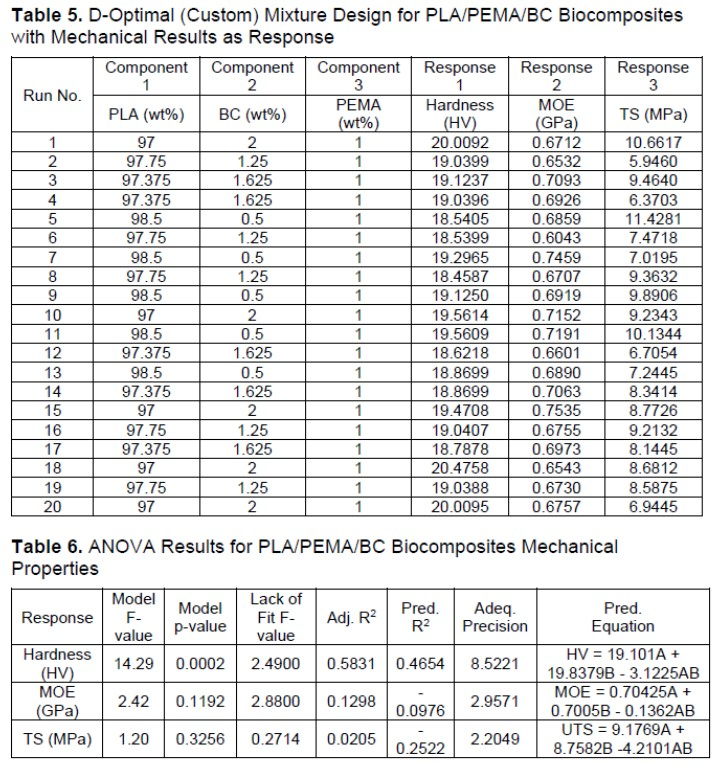Comparing the adequacy between the ANOVA of PLA/BC and PLA/PEMA/BC, the model and predicted values for PLA/PEMA/BC were slightly more reliable because of the noise level and significance of the ANOVA values. In terms of R2 values and adequate precision, the design expert guidelines, as supported by other studies, elaborated that an R2 closer to the value of 1.000 contributed to a model which was reliable and could predict the responses satisfactorily (Homkhiew et al. 2014; Radfar et al. 2020).

The plotted graphs of the PLA/PEMA/BC biocomposites predicted versus actual values generated by the mixture design were as shown in Figs. 4 to 6. In addition, the HV plot for the PLA/PEMA/BC biocomposite had a slightly better fitting model compared to the MOE and TS plots, similarly as the PLA/BC plots did.

Generated optimum mixture content were as follows: PLA/BC mixture = PLA (98 wt%) and BC (2 wt%); PLA/PEMA/BC mixture = PLA (97.75 wt%), PEMA (1 wt%), and BC (1.25 wt%).